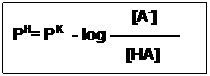# What is Henderson-Hasselbalch Equation? (Basic Concept)

The Henderson-Hasselbalch equation provides a general solution to the quantitative treatment of acid-base equilibrium in a biological system. This article explains how to derive the Henderson-Hasselbalch Equation.

Buffers are the mixture of weak acids and their salts of strong bases (or) the mixture of weak bases and their salts of strong acids. Simply, Buffers are an important concept of Acid-Base Chemistry. Buffers help to maintain a normal pH of the biological systems. When acid (or) alkali has added the pH of the solution changes in the absence of buffers.

## How buffers act?

### (Henderson-Hasselbalch Equation)

Buffers act as ‘Shock absorbers” against sudden changes of pH by converting injurious strong acids and bases into harmless weak acid salts.

HA –>  H+ + A

BA –>  B+ + A

If a buffer solution is composed of weak acid HA and its salt BA, they ionize as follows:

On addition of an Alkali, We shall have,

Na+ + OH + H+ + A–  –>  NaA + H2O

H+Cl + B+ + A —> HA + BCl

Example: Sodium acetate (CH3COONa+) + Acetic acid (CH3COOH)

C H3 COOH    +     C H3 COONa+     +     HCl

–>   C H3 COOH      +      C H3 COOH     +     NaCl

(Weak acid)

C H3 COO Na+    +    C H3 COOH     +     NaOH

–>      C H3 COO Na+    +     C H3 COO Na+    +    H2O

## Henderson-Hasselbalch Equation

The pH of a solution containing a weak acid is related to its acid dissociation constant. The relationship can be stated in the convenient form of the “Henderson-Hasselbalch equation”, derived below:

A weak acid, HA, ionizes as follows:

HA      –>    H+   +    A

The equilibrium constant for this dissociation is written as follows:

[H+] [A]

K=——————

[HA]

Cross multiply:

[H+][A] = K[HA]

Divide both sides by [A]

[HA]

[H+] = K————–

[A]

Take the log of both sides:

[HA]

log [H+] = log     K —————

[A]

[HA]

= logK + log ———

[A]

Multiply through by –1:

[HA]

-log [H+] = -log K – log ———-

[A]

Substitute pH and for –log [H+] and –logK, respective then:

[HA]

PH= PK – log ———

[A]

Then, to remove the minus sign, invert the last term:The Handerson-Hasselbalch equation is an expression of great predictive value in protonic equilibria.

For example,

1. When an acid is exactly half-neutralized, [A] = [HA] under these conditions,

[A]                               1

PH= PK – log ————- = PK + log —– = PK+ 0

[HA]                           1

Therefore, at half neutralization, PH = PK.

2. When the ratio [A-] /[HA] = 100: 1

[A]

PH= PK – log ————-

[HA]

PH      = PK – log  100/1  =   PK + 2

3. When the ratio [A-] / [HA] = 1:10,

PH=    PK – log 1/10        = PK + (-1)

If the equation is evaluate at several ratio of [A-] / [HA] between the limits 103 and 10-3 and the calculated pH values plotted, the result obtained describes the titration curves for a weak acid.

See this Video Tutorial Henderson-Hasselbalch Equation:

### 3 thoughts on “What is Henderson-Hasselbalch Equation? (Basic Concept)”

1.It’s surprising to find on biochemden.com a resource so
We will note your page as a benchmark for What is Henderson-Hasselbalch Equation? (Basic Concept).

We also invite you to link and other web resources for equations.

Thank you ang good luck!

2.3.